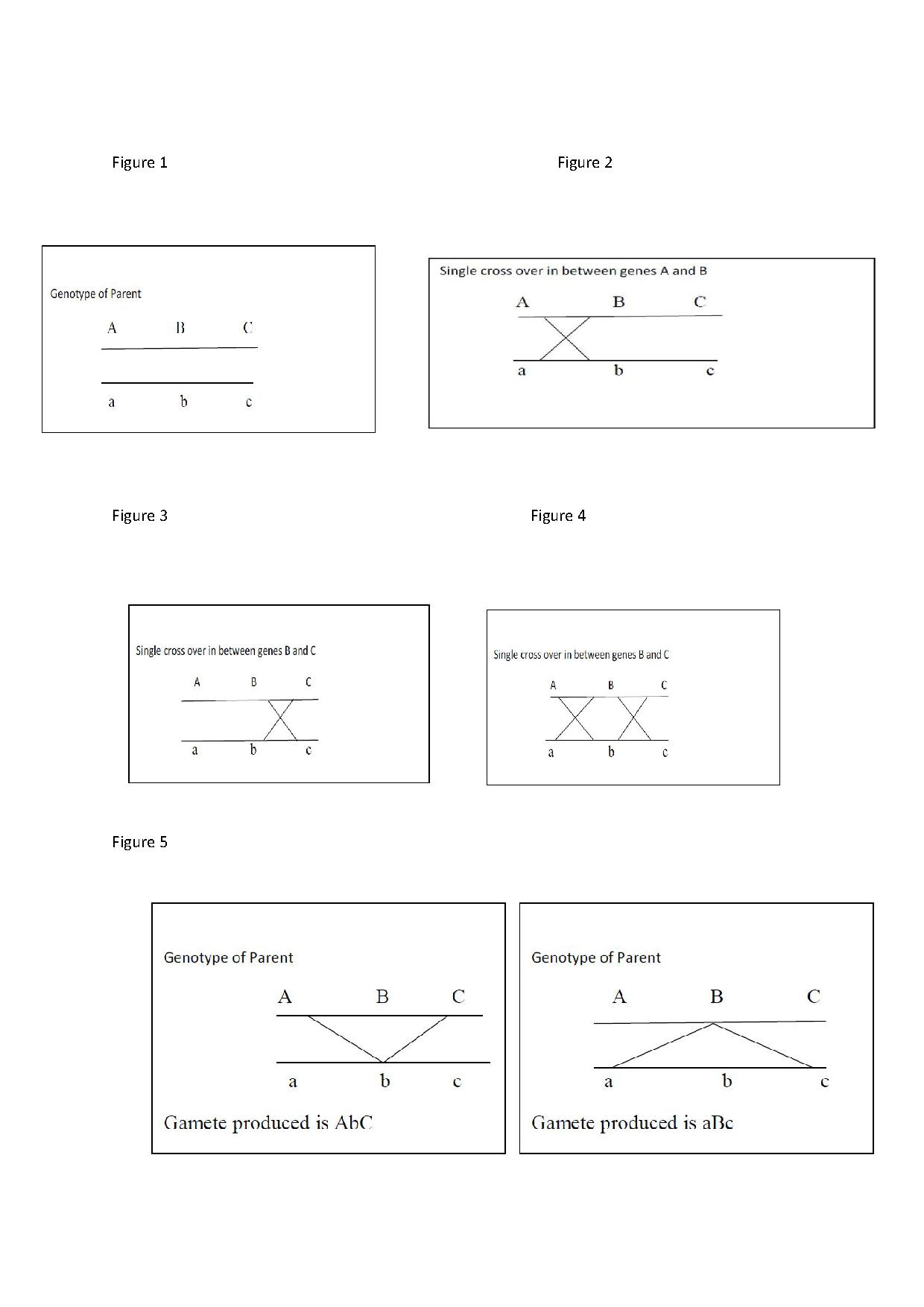CSIR NET June 2017

# How to solve Genetics problemsOct 03,2015

To solve genetics problem what you need is conceptual clarity and some tips and tricks which you can develop by practicing many problems daily. At least 5-6 question are asked in each CSIR exam from ‘C’ section. We will discuss some tricks to solve problems.

Most of the problems from linkage or recombination frequency are actually based on method of drawing the gametes from parent’s genotype.

Gametes can be obtained by two ways

1. Without recombination (lack of crossover event in meiosis)
2. With recombination (single crossover or double crossover events )

The parents homozygous for a particular gene will produce all the gametes which are identical.

e.g. AABB genotype parent will produce gamete as ‘AB’(either with recombination or without recombination)

Parent with aabb will produce gamete as ‘ab’ (either with recombination or without recombination)

The parents which are heterozygous for the genes can produces genetically different games depending upon number of genes in heterozygous state.

e.g. AaBb genotype parent will produce gamete as ‘AB’ and ‘ ab’ without recombination, and ‘Ab’ and ‘aB’ by recombination

Total number of different gametes can be calculated by using formula 2n  where n is total number of genes present in heterozygous state

e.g 1) AaBb here two genes are in heterozygous State

Total no of gametes =2n =2*2 =4 (AB, ab, Ab, aB)

1. AABb  here only one gene is in heterozygous state

Total no of gametes =2n =2*1 =2(AB, Ab)

1. AaBbCc  here three  genes are in heterozygous State

Total no of gametes =2n =2*2*2 =8 (ABC, abc, Abc, aBC, ABc, abC, AbC, aBc)

Drawing gametes from trihybrid parent

Consider the genotype of parent AaBbCc

First step draw two lines one below another (indicating pair of homologous chromosomes) and place one gene on first line and its allele immediately below on second line(indicating alleles of the same gene occupy  same locus on  pair of homologous chromosome ) (Figure 1)

Now the gametes can be drawn in two ways

1. Without recombination will involve two gametes as ABC  and abc (obtained by reading two lines horizontally)
2. The another way is by single crossover in between two genes A and B (Figure 2)

Moving across the cross line we can get two gametes as Abc and aBC

If single cross over occurs in between genes B and C (Figure 3)

Moving across the cross line we can get two gametes as ABc and abC

If a double cross over occurs in between A and B and B and C (Figure 4)

By moving across the line we get two types of gametes AbC, aBc (Figure 5)

Therefore from parent having genotype AaBbCc can produce 8 different types of gametes.

But whereas parents having genotype aabbcc can produce only one type of gamete as abc.

By using this method various problems on gene mapping by linkage and test crosses can be solved.

Return